This page has been robot translated, sorry for typos if any. Original content here.

 Start section Production, Amateur Amateur Radio Model aircraft, model aircraft Useful, entertaining Tricks to master Electronics Physics Technology Inventions Secrets of the cosmos Secrets of the Earth Secrets of the Ocean Tricks Section Map The use of materials from the site is permitted provided the link (for sites - hyperlinks)
 Navigation: => Home / Physics / Discoveries /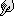# TO THE QUESTION ABOUT WAVE INTERFERENCE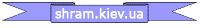## Stetsovich V.I.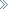Leave a comment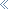The physical processes during the formation, propagation, interference and diffraction of acoustic waves are considered.

It is shown that the energy density, which is carried by the wave, is proportional to the amplitude and frequency of the source oscillations, and when the waves interfere, the energy of the resulting oscillation is equal to the sum of the energies of the folding waves.

Modern wave theory explains a very wide range of wave processes observed in nature. And, nevertheless, the analysis of interference effects leads to results that contradict the conclusions of the wave theory.
In accordance with generally accepted ideas, when adding at some point of observation two coherent waves propagating in one direction: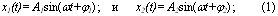the harmonic oscillation of the same period will again be obtained: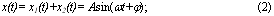amplitude A and initial phase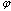the resulting oscillation of which is determined from the vector diagram ( Fig. 1 ):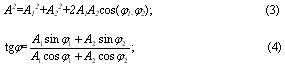Since the wave energy density is proportional to the square of the amplitude of what oscillates, when interference occurs, the energy of the resulting oscillation is not equal to the sum of the energies of the folding waves and varies from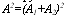at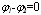at the point of interference maximum to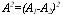at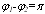at the points of the interference minimum.
In the simplest case, the equality of the amplitudes of the interfering waves: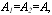, the amplitude of the resulting oscillation varies from zero at the minimum to 2A at the maximum, and the corresponding intensities from 0 to 4A 2 .

The damping of vibrations in some places and their amplification in others are not associated with any transformations of the vibrational energy. Thus, in the case of interference, the matter boils down, as it were, only to the redistribution of the oscillation energy and a change in the direction of its transfer, but the law of conservation of energy is strictly fulfilled .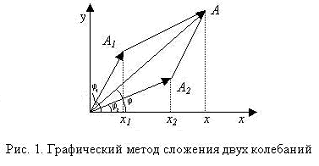The distribution of the energy density of a sound wave along its direction of propagation is shown in Fig. 2 . It can be seen from the figure that, in the condensation region and in the rarefaction region, equal energy flows are transmitted by the wave, therefore, when two waves interfere, and at the point of the interference maximum (m. A , Fig . 3 ), when the amplitudes of pressures and velocities are added, and at the point of interference minimum (m. O , Fig . 3 ), when the region of condensation of one wave overlaps with the rarefaction region of the other, two regions of the waves are transferred, which carry the same energy, which must be equally recorded by the instruments. However, energy is not fixed at the points of the interference minimum.

The redistribution of energy to the points of the interference maximum is possible only if the interference changes the direction of energy transfer by the wave. Considering in this case a longer path and a constant velocity of wave energy propagation in the medium, it would be possible to fix a slowdown in the wave energy propagation in the interference region. Since this is not the case, it should be assumed that in the interference region, the energy is transferred by the wave at a speed greater than the speed of wave propagation in the medium, which is obviously unrealistic.

During propagation, the waves pass through one another, without affecting each other at all. Similarly, when passing points with zero amplitude of oscillations during interference (m. O , Fig . 3 ), two coherent waves propagate further without any changes, and at the maximum point (m. A , Fig . 3 ), the amplitudes of oscillations of waves are observed without any or time delays. Consequently, the waves transfer energy through the points of the interference minimum and there is no redistribution of energy to the points of the interference maximum.
Problems arise in explaining the interference of electromagnetic waves. An electromagnetic wave is a combination of variable electric and magnetic fields propagating in space and, since two coherent waves are superimposed at an interference minimum point, the electric field strength is constant to zero, the wave propagation further without alternating fields ( E and H ) through the interference minimum point . It is also not clear for what reasons, after passing the interference minimum, the amplitude of the electromagnetic wave increases.

In the case of corpuscular interpretation of the interference phenomenon of electromagnetic waves, it is impossible to explain how an energy release is observed at the interference maximum, which exceeds the sum of the photon energy, and it is completely incomprehensible how and where the energy and momentum are transmitted by photons at the interference minimum and where they occur after its passage.

The essence of the wave process is the transfer of oscillation energy from one point of space to another, etc. What is the mechanism of energy transfer through areas of space, where measurements show the absence of waves and, accordingly, energy transfer?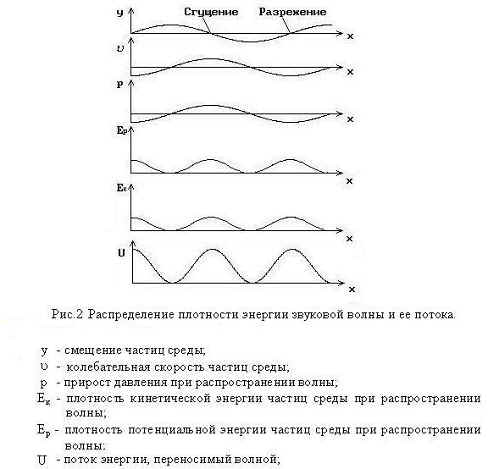To explain these phenomena, it seems necessary to consider in more detail the processes occurring during the occurrence and propagation of waves. Since the basic laws of wave motion are the same, we will consider the case that is easiest to clearly explain - the propagation of a sound wave in an ideal gas.

When analyzing the processes occurring during the propagation of a sound wave in an ideal gas, we assume that:

• gas molecules have the same mass;
• in collisions of molecules with each other - the interaction is elastic, according to the laws of the collision of balls;
• two molecules collide as a result of movement; simultaneous collisions of three or more molecules are unlikely;
• particles experience only frontal collisions, which only lead to a change in the direction of the colliding molecules and do not change the direction of their velocities to any other angles;
• molecules can move in the direction of the x, y, z coordinate axes, and out of 1/3 of the total number of molecules moving along the x axis , half move in the direction of the screen, which will be the source of disturbances, and the other half from it;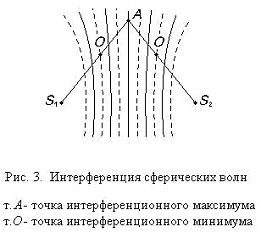Suppose that under the action of an external force, the screen moves with speed u in the direction of the x axis ( Fig . 4 ). An arbitrarily chosen gas molecule, moving towards the screen with the velocity “ -u0 ”, after the impact will have the velocity u0 + u . In this case, the fact that the temperature of the medium and the screen are the same is taken into account, and in the process of interaction, the molecule is first adsorbed on the screen surface and flies out after some time, having a velocity of u0 relative to the screen, and u0 + u relative to the external observer. If we consider the process of interaction of a molecule with a moving screen as an elastic impact of balls, then the speed of the molecule will be u0 + 2u and all the formulas given below will differ only by coefficients.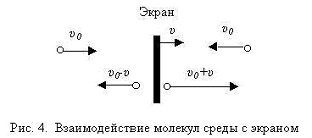In any case, the momentum and kinetic energy of the molecules increase, which leads to an increase in the internal energy of the gas, as compared with the unperturbed state.

On the opposite side of the screen, after colliding with it, the speed of the molecules will decrease: u0 -u , and the internal energy of the gas will be less than the initial, unperturbed state.

The resulting perturbation of physical parameters in the medium, as a result of the interaction between particles according to the law of conservation of the impulse of a closed system, will propagate at a speed c , and, moving from the screen, half of the particles moving along the x axis transfer the energy of the perturbation, and after colliding with particles moving towards, in the opposite direction, the energy corresponding to the energy of the particle in the unperturbed state of the medium ( Fig. 5 a, b ).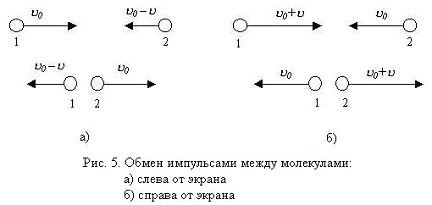As the screen moves, the particle density changes.

Taking into account that u «c , in the condensation area to the right of the screen :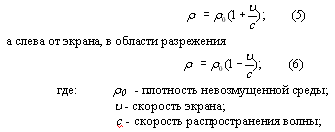In this case, the kinetic energy of particles in a unit volume, carrying the energy of perturbation in the direction away from the screen, is: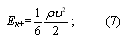and the kinetic energy transferred at the same time by the other half of the particles in the opposite direction, to the screen: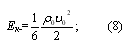The difference between (7) and (8) determines the magnitude and direction of the energy perturbation carried by the wave and to the right of the screen where the thickening impulse propagates, the wave energy density is: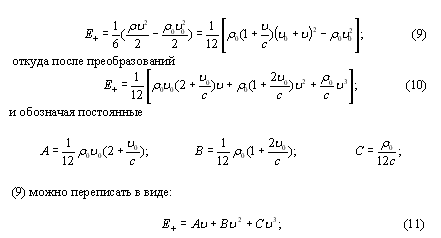Similar calculations will find the energy density, which is transferred by the rarefaction wave to the left of the screen: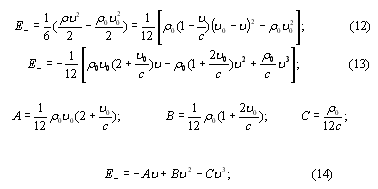Since during the propagation of a wave, the concentration of particles of the medium changes, i.e. the average distance between them means that the potential energy of the selected volume of the medium is also changing. In the classical theory, the wave energy consists of the kinetic energy of the particles of the medium making the oscillations and of the potential energy of the elastic deformation of the medium, and the densities of the kinetic and potential energies are equal at any time and at any point of the medium ( Fig . 2 ).
The potential energy of interaction of two particles, depending on the distance between them, has approximately the form shown in Fig.6 . Under normal conditions ( p = 1 atm , and T = 200C, r ~ 10r0 ), the distance between air molecules is r »rо, therefore, when a sound wave propagates in such a medium, the potential energy of the medium increases in the rarefaction region, and in the thickening region (unlike classical theory) - decreases. Considering, however, the fact that for gas molecules under given conditions Ek »Ep , a change in the potential energy of interaction of molecules, with the propagation of acoustic waves in the atmosphere, compared with a change in their kinetic energy can almost be neglected and the gas can be considered as ideal.

The speed of the screen u экрана c and u и u0 . Then, neglecting in (10) and (13) terms proportional to u2 and u3 , we can fairly accurately assume that in the general case, in vector form, the energy density carried by a plane wave: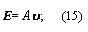where the vector u determines the magnitude of the wave energy and the direction of its propagation.
If the screen oscillations are harmonic: x = Xsinwt and its speed is u = Xw coswt , then the value of the energy density transferred by the wave is fundamentally different from the values ​​of energy determined by the classical formulas [ 4, p.363 ]: the wave energy is proportional to the density of the medium, the speed of the chaotic thermal molecular motion, amplitude and frequency, and not squares of the amplitude and frequency of the source.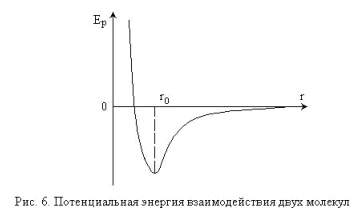The energy components proportional to u2 and u3 make a significant contribution to the wave energy at large amplitudes and oscillation frequencies of the source, which, for example, when acoustic waves propagate in a medium, propagate non-linear effects.

Acquired by particles in a unit volume of the medium, the pulse during the propagation of a condensation wave is: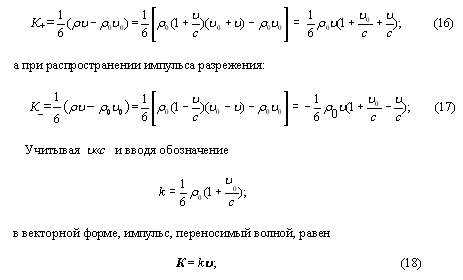Since the direction of the screen speed u changes every half-period, every half-period (unlike the classical theory) the direction of the transferred wave of momentum and energy also changes. The instantaneous distribution of internal energy in the medium and the direction of energy transfer during the propagation of a plane wave along the x axis at some time point are shown in Fig. 7 , where E is the direction of energy transfer by the wave that is emitted by the source, and Еs is the direction of energy transfer due to redistribution of the internal energy of the medium (the screen is located at the origin of coordinates). The instantaneous value of the energy transmitted by the wave E (x, t) at the point x at the moment of time t (Fig. 8) is equal to: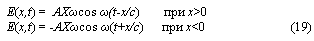moreover, a positive value of E means that the direction of energy transfer and wave propagation coincide, negative - the energy is transferred (unlike the classical theory) in the opposite direction.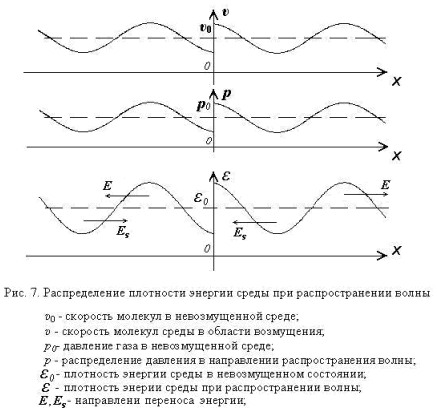In practice, when registering an acoustic wave and its parameters, the sound pressure is determined, and microphones are used as receivers of acoustic oscillations, which in one way or another monitor the pressure fluctuations of the medium during the passage of the wave and convert them into electrical oscillations, and in classical physics the wave intensity is proportional to the square of the sound pressure .
It is known that gas pressure in an unperturbed medium: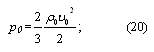In this case, the change in the pressure of the medium on the membrane of the microphone during the propagation of a sound wave caused by a change in the density and velocity of the particles is determined from the expression: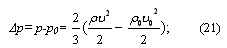Noting that the value in brackets is proportional to the energy carried by the wave, we conclude that the density of the wave energy, and, accordingly, the sound intensity, is proportional to the amplitude of the sound pressure, and not to its square: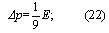The reason for all these differences is that in the classical theory, when calculating the wave energy, the fact that the particles of the medium already have an initial velocity and kinetic energy before the appearance of a disturbance in the medium is ignored ( Fig. 7 ).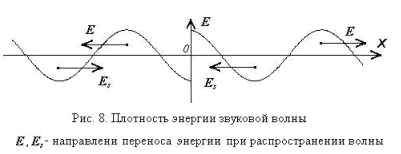Fundamentally different from the conventional and explanation of the process of wave interference.

In the classical version, if two coherent waves are excited in a homogeneous and isotropic medium, then in an arbitrary point of space the waves are superimposed according to the principle of superposition: each point (molecule) of the medium, where two or several waves arrive, simultaneously participates in oscillations caused by each wave separately . To determine the motion of a particle, the motion of a medium particle in each wave is found separately, and then these movements are summed up ( Fig . 1 ).

In this variant: two particles, each of which carries a disturbance caused by two different sources of oscillations, in the case of interaction exchange impulses: the first after the collision will transfer to the next collision the impulse transmitted to it by the second particle and vice versa: the second particle will transfer the impulse transmitted to it first i each particle takes part in the transfer of disturbance from only one source ( Fig. 5 ). The interaction option presented in Fig. 1 is possible except in the case of simultaneous impact of two particles in the third, but, according to the initial conditions, such an event is unlikely. Therefore, for particles of a medium in which several waves propagate, the principle of superposition is unacceptable: each particle participates in the transfer of momentum and energy only from one source, or: the perturbation from different sources is transferred by different particles.

As a result, it can be argued that if interference occurs, the waves propagate without affecting one another, there is no redistribution of energy flows and other interaction as a result of wave superposition, and the total energy density at the observation point is equal to the total energy density of the interfering waves: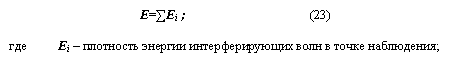If two waves with the same amplitudes propagate in the same direction, at the points of the interference maximum, the amplitude of the sound pressure doubles, which means that the energy densities of the folding waves are added together ( Fig . 9a ). At the points of the interference minimum, when the region of condensation of one wave with the vacuum region of another is superimposed, the signal is not fixed, because in this case the directions of energy and momentum transfer by interfering waves are opposite, and, with equal amplitudes of oscillations, their total effect on the microphone membrane is zero ( Figure .9b ). Therefore, when adding waves, it is necessary to take into account not only the amount of energy and momentum carried by the wave, but also the direction of their transfer.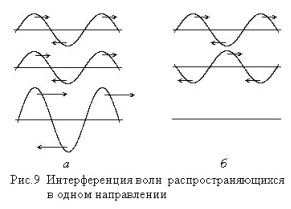Similar processes occur in the standing wave.

It is known that when two coherent, identical amplitudes interfere with waves traveling in opposite directions, the resulting wave motion is called a standing wave. A standing wave is characterized by the presence of nodal points (interference minimum points), the amplitude of oscillations of which is zero, particles of the medium at these points do not move at all, through these points (according to classical ideas) there is no energy transfer and, in general, energy does not spread along the standing wave, only particles between nodes oscillate and exchange energy .

In this interpretation, the explanation is different.

In the so-called the nodal points of the particle, moving continuously, in one direction transfer the energy of the wave running in the same direction before colliding with particles that transfer the energy of the wave propagating in the opposite direction. After a collision and an exchange of pulses in the opposite direction until the next collision, the particles transfer a momentum of a wave propagating in the same direction. So, periodically changing the direction of motion and exchanging pulses, the particles alternately transfer the energy of both waves, so they propagate each in their own direction without affecting each other. With the help of receivers of sound waves, for example a microphone, with small amplitudes of oscillations, counter-flowing energy and momentum flows through the nodal points of the standing wave and the points of the interference minimum with the interference of waves with equal amplitudes of oscillations cannot be detected, since their effect on the receiver is the same in magnitude but opposite in direction and as a result is zero. But, nevertheless, at the points of the interference minimum, the waves do not “quench” each other, and the state of “excited” particles at nodal points: speed, energy, and particle density differs from the corresponding values ​​in an unperturbed medium.

Until now, when considering the issues of wave propagation, the fact that particle collisions lead to a change in the direction of the velocity of the colliding particles at some angles different from the initial direction of wave propagation has not been taken into account. As a result of these deviations, the wave energy carried by particles can also propagate at an angle to the original direction. On the screen area (wave source), these energy flows (due to the randomness of the processes of movement and scattering of molecules during collisions) will be mutually compensated on average, and only at the lateral boundaries of the plane wave will the wave energy propagate away from the original direction. Similar processes will occur when a wave encounters an obstacle, which is the cause of wave diffraction.

Similar processes of transmission or diffusion of the amplitude of oscillations along the wave front (across the beam) are also observed during wave propagation, when the amplitude along the wave front changes.

The wave transfers momentum and energy from one place of space to another, and it is considered that all particles of the medium involved in energy transfer fluctuate around the equilibrium position all the time. From (10), (13) and (16), (17) it can be seen that, when a longitudinal wave propagates, the impulse and energy transferred to the positive half-wave exceed the transferred impulse and energy in the negative half-wave by the values ​​respectively: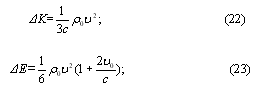which leads to the transfer of the substance of the medium by the sound wave, and at large amplitudes leads to the appearance of a constant flow in the form of a so-called. acoustic currents.

## FINDINGS

1. The energy carried by the wave is proportional to the amplitude and frequency of oscillations of the source, and not to their squares.

2. At interference, the energy of the resulting oscillation is equal to the sum of the energies of the folding waves.

3. The energy transferred by the wave of the perturbation is characterized not only by the magnitude, but also by the direction of its transfer and during the propagation in the medium of a harmonic wave, the direction of transfer changes every half-period.

## LITERATURE

1. G.S. Landsberg, Optics, Science, Moscow, (1976), p. 88

2. S.P. Strelkov, Mechanics, Science, Moscow, (1975), p. 481.

3. S.E. Khaykin, Physical foundations, Radyanska school, Kiev, (1966), p. 674.

4. V.Ye. Kuzmichyov, Laws and formulas of physics, Naukova Dumka, Kiev, (1989), p. 363.

print version
Authors: Stetsovich V.I.
Publication date 10.11.2006NEW ARTICLES AND PUBLICATIONSManufacturing technology of universal couplings for weld-free, threadless, flangeless connection of pipe sections in high-pressure pipelines (video available)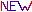Technology of refining oil and oil productsOn the possibility of moving a closed mechanical system due to internal forcesGlow of liquid in thin dielectric channelsThe relationship between quantum and classical mechanicsMillimeter waves in medicine. A New Look. IIM therapyMagnetic engineHeat source based on nasal units